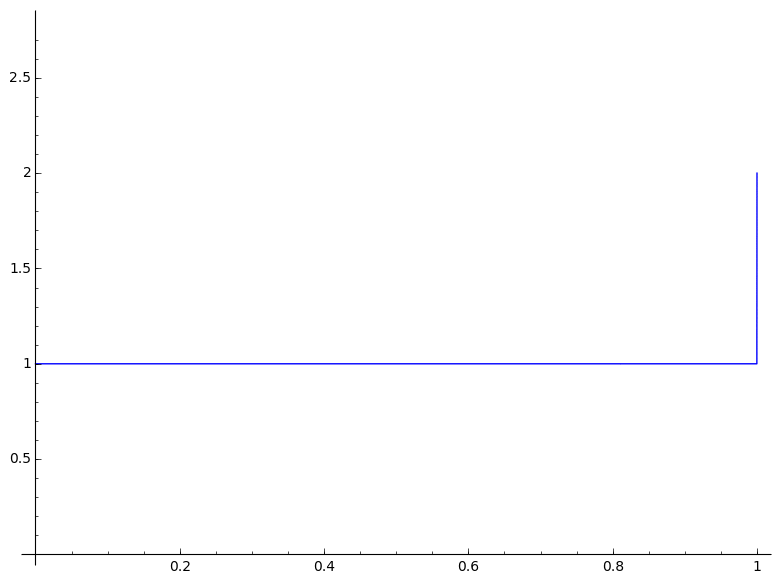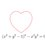# Euler's Number $(e)$ - Infrequently Asked Questions• What do I need to know to read this?

Some basic idea of differentiation. Here is a (very) informal introduction.

• So, What is $e$?

$e$ is the unique real constant such that the differential equation $\frac{dy}{dx} = ky$ has a solution of the type $y=Ce^{kx}$, which is equivalent to saying that $e = \lim_{n \to \infty}{\left ( 1+ \frac{x}{n} \right ) ^ {\frac{n}{x}}}$

• Why should I care?

Well, these functions pop up all the time. Here is a classical example:

Say, I have a culture of bacteria which is continuously replicating. It is obvious(why?) that the rate of growth of the culture is proportional to the size of the culture itself.

Let us now try to express the size of the culture ($y$) as a function of time($t$).

Using what we just pointed out, we have:

$\frac{dy}{dt} \propto y \\ \frac{dy}{dt} = ky \quad \text{where k is the rate constant}$

Compare that with the equation in the definition!

So, we have $y(t) = Ce^{kt} \quad \text{where C was the initial size}$

• I am not very fond of bacteria. Nor do I understand why you emphasized continuously over there.

Well, you must know of compound interest.

Say, my principle was $P$, rate $R$ and I compound anually.

• At the end of the first year, I have $(P + PR) = P (1+R)$
• At the end of the second year, I have $P(1+R) + R(P(1+R)) = P(1+R)^2$
• At the end of the tth year, I have $P(1+R)^t$

Okay, now what if I compound half yearly? Wouldn't it be better because I'd have more money that is compounding during the mid-year?

Yeah, that is correct.

• At the end of the first half-year, I have $(P + P\frac{R}{2} = P (1+\frac{R}{2})$
• At the end of the year, I have $P(1+\frac{R}{2}) + \frac{R}{2}(P(1+\frac{R}{2})) = P(1+\frac{R}{2})^{2}$
• At the end of the tth year, I have $P(1+\frac{R}{2})^{2t}$

Well, if I compounded $n$ times an year, I'd be getting $A = P(1+\frac{R}{n})^{n t}$

I could keep compounding continuously too, which would mean the amount is never at rest.

That is $A_{cont} = \lim_{n \to \infty} P(1+\frac{R}{n})^{n t} \\ = \lim_{n \to \infty} P(1+\frac{R}{n})^{\frac{R n t}{R}} \\ = P \lim_{n \to \infty} (1+\frac{R}{n})^{\frac{n}{R}{Rt}} \\ = P e^{Rt}$

• Pretty Cool! Could you show this graphically?

Yes! Look at the top of the note!Sage Code:

 1 2 3 4 a = lambda n,t: (1+(1/n))^(n*(floor(n*t)/n)) contd = [plot(lambda t: a(n,t), (0,1),ymin=0,ymax=2.8) for n in xrange(1,31)] anim = animate(contd) anim.show() 

• You said $e$ is a real number. How do I compute it's value then?

Here is one (simple) way:

Recall the second second definition.

$e = \lim_{n \to \infty} {\left ( 1+ \frac{x}{n} \right ) ^ {\frac{n}{x}}} \\ \implies e^x = \lim_{n \to \infty}{\left ( 1+ \frac{x}{n} \right ) ^ n } \\ = \lim_{n \to \infty}{\left ( 1+ \frac{1}{n} \right ) ^ n} \\ = \lim_{n \to \infty}\sum^{n}_{k=0} {n \choose k} \frac{1}{n^k} \\ = 1+1+\lim_{n \to \infty} \sum_{k=2}^n\frac{n(n-1)(n-2)\cdots(n-(k-1))}{k!\,n^k} \\ = 1+ 1+\frac{1}{2!}\left(1-\frac{1}{n}\right)+\frac{1}{3!}\left(1-\frac{1}{n}\right)\left(1-\frac{2}{n}\right)+\cdots \\ = 2 + \frac{1}{2!} + \frac{1}{3!} + \frac{1}{4!} + \cdots$

• I still do not see why that is the unique number as promised in the first definition.

Here you go:

Proposition Let $f : \mathbb{R} \to \mathbb{R}$ be a differentiable function such that $f(0) = C$ and $f'(x) = f(x)$. Then it must be the case that $f = Ce^x$.

Proof Let $g(x) = f(x) \frac{e^{-x}}{C}$. Then

$g'(x) = -f(x) \frac{e^{-x}}{C} + f'(x) \frac{e^{-x}}{C} = (f'(x) - f(x)) \frac{e^{-x}}{C} = 0$

by assumption, so $g$ is constant. But $g(0) = 1$, so $g(x) = 1$ identically.

Hence, $1 = f(x)\frac{e^{-x}}{C} \\ \implies f(x) = Ce^x$

• Why is the logarithm base $e$ termed natural?

Because it is!

You have seen how $e$ relates to every exponential growth scenario, now it is obvious why the natural logarithm relates to time.

The growth velocity of bacteria population varies with it's size. So, the time taken to grow by an amount would vary inversely with it. Hence, we have $\frac{d}{dx}\ln x = \frac{1}{x}$.

More precisely, our bacteria culture which grew as a function of time as $y(t) = Ce^{kt}$ is of a specific size $y$ at time $t = \frac{\ln(\frac{y}{C})}{k}$

What is important is to observe that $\frac{y}{C}$ is the amount by which it grew and $k$ is the rate constant.

• What does it mean to say that $e^{i \pi} + 1 = 0$ ?

That is one of the most beautiful equations of all time!

$e^{i x} = 1 + i x + \frac{(ix)^2}{2!} + \frac{(ix)^3}{3!} + \frac{(ix)^4}{4!} + \cdots \\ =1 + i x - \frac{x^2}{2!} -\frac{ix^3}{3!} +\frac{(x)^4}{4!} + \cdots \\ = \left(1- \frac{x^2}{2!} + \frac{x^4}{4!}+\cdots\right) + i\left( x- \frac{x^3}{3!}+\frac{x^5}{5!}\cdots\right) \\ = \cos x + i \sin x$

So, $e^{i \theta}$ is actually an unit vector on the complex plane with argument $\theta$. In general, $R e^{i \theta}$ would be a complex number of modulus $R$ and argument $\theta$.

Clearly, $e^{i \pi} = -1 \implies e^{i \pi} + 1 = 0$.

In essence, $e$ forms the basis of exponentiation in the complex plane. Here, we use it to show that $i^i$ is real!

• You still did not answer my question!

I probably do not know what your question is. Feel free to ask me in the comments.6 years, 7 months ago

This discussion board is a place to discuss our Daily Challenges and the math and science related to those challenges. Explanations are more than just a solution — they should explain the steps and thinking strategies that you used to obtain the solution. Comments should further the discussion of math and science.

When posting on Brilliant:

• Use the emojis to react to an explanation, whether you're congratulating a job well done , or just really confused .
• Ask specific questions about the challenge or the steps in somebody's explanation. Well-posed questions can add a lot to the discussion, but posting "I don't understand!" doesn't help anyone.
• Try to contribute something new to the discussion, whether it is an extension, generalization or other idea related to the challenge.

MarkdownAppears as
*italics* or _italics_ italics
**bold** or __bold__ bold
- bulleted- list
• bulleted
• list
1. numbered2. list
1. numbered
2. list
Note: you must add a full line of space before and after lists for them to show up correctly
paragraph 1paragraph 2

paragraph 1

paragraph 2

[example link](https://brilliant.org)example link
> This is a quote
This is a quote
    # I indented these lines
# 4 spaces, and now they show
# up as a code block.

print "hello world"
# I indented these lines
# 4 spaces, and now they show
# up as a code block.

print "hello world"
MathAppears as
Remember to wrap math in $$ ... $$ or $ ... $ to ensure proper formatting.
2 \times 3 $2 \times 3$
2^{34} $2^{34}$
a_{i-1} $a_{i-1}$
\frac{2}{3} $\frac{2}{3}$
\sqrt{2} $\sqrt{2}$
\sum_{i=1}^3 $\sum_{i=1}^3$
\sin \theta $\sin \theta$
\boxed{123} $\boxed{123}$

Sort by:

@megh choksi ,@Amrita Roychowdhury, @Sneha Saha : Please have a look

- 6 years, 7 months ago

solution means a root of an equation according to me , do you agree?

If yes , then you explained in the earlier note that $\dfrac{dy}{dx}$ means rate of change of a quantity with respect to other or a tangent to the curve.

Now how can a magnitude( rate of change of a quantity with respect to other) have a root - seems really odd!

or how can a tangent(which represents a direction) can have root?

- 6 years, 7 months ago

Solution is a very general term. It does not have a strict definition.

No, by solution of a differential equation, a root, i.e, a number that satisfies the equation is not what is meant.

The solution to a differential equation, is an analytical equation which upon differentiating gives back the equation.

Here is what comprises an analytical expression:analytic

The solution to $\frac{dy}{dx} = ky$ can be $y = Ce^{kx}$ because differentiating this in gives the differential equation.

- 6 years, 7 months ago

Thanks sir

- 6 years, 7 months ago

There's a typo in your comment. It should be $y=Ce^{kx}$

- 6 years, 5 months ago

Fixed. I missed out the {}

- 6 years, 5 months ago

I thought so. Typing raw $\LaTeX$ code quickly sometimes makes you miss the brackets. :D

- 6 years, 5 months ago

Very informative . Helped to brushen up my concepts .

- 6 years, 6 months ago

I take very high offense on how you called the first way of calculating $e$ "lame".

- 6 years, 7 months ago

It's not that lame but there are faster converging methods.

- 6 years, 7 months ago

True.

- 6 years, 7 months ago

But $e^{x}$ is one of the most renowned Taylor series simply because $e^{ix}= \cos x+ i \sin x$ :)

- 6 years, 7 months ago

"Proof" that $e^{\pi i}\approx-1$

$e\approx1.000001^{1000000}$

$1.000001^x\approx1+.000001x$ for small x (try it)

$e^{\pi i}\approx(1.000001^{1000000})^{\pi i}$

$=(1.000001^{\pi i})^{1000000}$

$\approx(1+.000001\pi i)^{1000000}$

$\approx-1.000005+.00000000001i$

$\approx-1$

Therefore, $e^{\pi i}\approx-1$. Somehow. I guess.

(From a correspondence: "Ooh, nice! How did you get from (1+.01πi)^100 to -1 + junk?" "I multiplied it by itself 1000000 times.")

- 6 years, 3 months ago

Before anyone says that's not a real proof: You don't say?!

- 6 years, 3 months ago

I think the question is, If this zigzag function is continualy shrinks by a factor k, what will be the its length at infinity?

- 5 years, 9 months ago